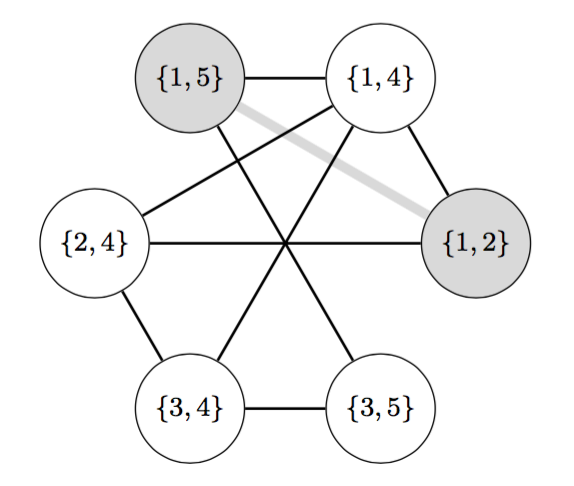# Occurrence graphs of patterns in permutations

### Involve, Volume 12 (2019), Number 6, 901-918We define the occurrence graph of a pattern p in a permutation as the graph with the occurrences of p in the permutation as vertices, and edges between the vertices if the occurrences differ by exactly one element. We then study properties of these graphs. The main theorem in this paper is that every hereditary property of graphs gives rise to a permutation class.

This paper started as the BSc thesis of Bjarni at the University of Iceland.